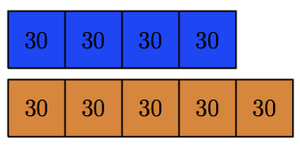# Evaluating Ratio Statements

Alignments to Content Standards: 6.RP.A.1

The ratio of the number of boys to the number of girls at school is 4:5.  There are 270 students at this school. For each of the following statements, explain whether the statement is true or false and why:

1. The number of boys at school is 4/5 the number of girls.
2. 4/5 of the students in the school are boys.
3. There are exactly 30 more girls than boys.
4. There are exactly 30 boys at the school.
5. 5/9 of the students in the school are girls.

## IM Commentary

The goal of this task is to assess student understanding of ratios.  The task offers five questions, some of which can be addressed using ony the given ratio, whereas others require knowledge of the total number of students.  It may be instructive to have students articulate the difference between these two types of questions.  Of course, by simple rewording of questions, many other questions are possible in this simple context, and it would be of value to have students grow familiar with a variety of phrasings.

The solution given here employs tape diagrams, one of the most concrete representations of ratios, which are particularly well suited for understanding and applying ratio concepts involving similar units.

One specific area of difficulty that may arise is the tension between students' prior experience with fractions representing a part to whole relationship and their new understanding of ratio as a relationship between parts or between the part and the whole.  Again, a tape diagram can help students understand both the part-whole relationship that exists (as targeted by the statement about the girls representing 5/9 of the school) and the part-part relationship that exists (as targeted by the statement about the total number of boys being 4/5 the total number of girls.)

The solution also presents ratio tables which summarize the information presented by the tape diagrams in a non-graphical manner.  Teachers will want to note which students tend to build out each iteration of the ratio table by skip counting and which students use more advanced strategies such as multiplying each row by a common factor.

## Solution

One way to answer each of these questions is to first determine from the given information how many boys and how many girls there are at the school. There are several ways to do this. One method would be to use a tape diagram:Here there are four blue squares representing boys and five orange squares representing girls. This picture represents the 4:5 ratio of boys to girls.  This tape diagram is already enough to answer parts (a), (b), and (e) of the question.

For (c) and (d), we need to know the actual number of students represented by each square.  To do this, notice that there are 9 squares total representing 270 students. This means that each square represents 270 $\div$ 9 = 30 students:Another way we could discover this would be with a ratio table:

Boys Girls Total Students
4 5 9
40 50 90
80 100 180
120 150 270

In terms of the tape diagram, the first row of the ratio table assigns a value of 1 to ?, the second row assigns a value of 10, the third row assigns a value of 20, and the third row a value of 30. The ratio table allows us to discover the final row of the table in steps as opposed to all at once. With this information we can answer all of the questions:

1. True.  The first tape diagram shows there are 4 equal groups of boys compared to 5 of the same equal groups of girls so the number of boys is 4/5 of the number of girls.
2. False. The fraction of students who are boys is not 4/5 but rather 4/9. Here the whole is all students. All of the students are represented by 9 equal groups, 4 of which are boys.
3. True. There are 30 more girls than boys. We can see this using the tape diagrams above. Alternatively, for each 5 girls there are 4 boys so this is one more girl than boy. The scale factor to go from 9 students to 270 students is 30. So the 1 extra girl, compared to boys, is scaled by 30 for the school, giving 30 more girls than boys.
4. False. There are not exactly 30 boys at the school. For this problem, 30 is the scale factor to go from 9 students to 270. Alternatively, it is the number of students in each group of the tape diagram. There are 4 of these groups of boys or 120 boys total.
5. True. The fraction of the students who are girls is 5/9. This is seen in the first tape diagram. There are 9 equal boxes representing all students and five of these boxes represent girls.